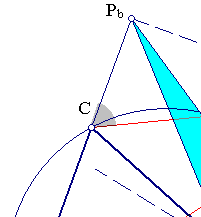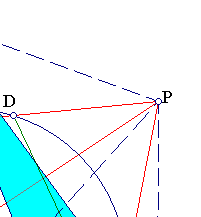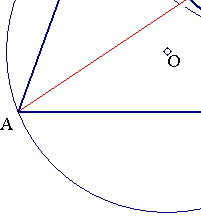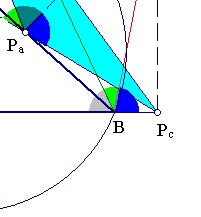##Area of pedal triangle of a point

Consider the triangle of reference ABC and a point P. The pedal triangle of P with respect to ABC is the triangle PaPbPc of the projections of P on the sides of ABC (see Pedal.html ).
 The area of the pedal triangle is
area(PaPbPc) = (R2-|PO|2)*sin(A)*sin(B)*sin(C)/2,
where R is the circumradius of ABC and O is the circumcenter (R2-|PO|2 is the power of P w.r. to the circumcircle).
 For P moving on circles concentric to the circumcircle, the pedal triangles have constant area and vice versa. In particular, for P on the circumcircle the corresponding area is zero, the three projection-points being on the Simson line of P.
 Denoting by (x,y,z) the trilinear coordinates of P, and considering signed (oriented) areas:
area(PaPbPc) = (1/2)*(sin(A)*y*z+sin(B)*z*x+sin(C)*x*y)
is a quadratic form in the trilinear coordinates.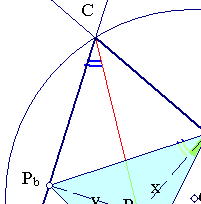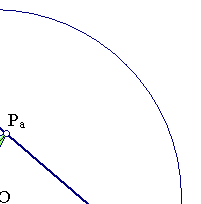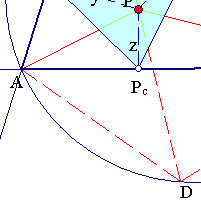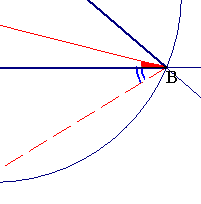The formula follows by extending PC to cut the circumcircle of ABC at D and observing that angle(PcPaPb) = angle(DBP). Also angle(PDB) equals angle A of the triangle and by the sinus theorem applied to triangle DBP we have: sin(<(DBP))/|PD| = sin(A)/|PB|.(*)
In addition, since APbPcP is cyclic and PA is a diameter we have |PA| = |PbPc|*sin(A) and analogous equations for the segments PB and PC.(**)
Now using (*) and (**) the area of the pedal triangle is:
area(PaPbPc) = |PaPb|*|PaPc|*sin(<(PcPaPb))/2 = |PC|*sin(C)*|PB|*sin(B)*sin(DBP)/2 = |PC|*|PD|*sin(A)*sin(B)*sin(C)/2
= (R2-|PO|2)*sin(A)*sin(B)*sin(C)/2.
 Follows immediately from .
 Follows by dividing the area of the triangle in the sum: area(PaPbPc) =area(PPbPc)+area(PaPPc)+area(PaPbP).

Remarks
 Formula (1) is general valid, even when point P is outside the triangle, provided we use oriented areas. Next figure illustrates the corresponding proof.
 By equating the expressions in (1) and (3) we get:
sin(A)*y*z+sin(B)*z*x+sin(C)*x*y = (R2-|PO|2)*sin(A)*sin(B)*sin(C). <==>
a*y*z+b*z*x+c*x*y = (R2-|PO|2)*a*b*c/(4*R2).
By taking P on the circle we get its equation in trilinears (see also CircumcircleInTrilinears.html ).
 The previous equation shows that the quadratic form f(x,y,z) = a*y*z+b*z*x+c*x*y, where a, b, c denote the lengths of the sides of the triangle, is positive inside the circumcircle, zero on the circumcircle and negative outside. Notice that (x,y,z) are not independent, but satisfy the equation a*x+b*y+c*z = 2*area(ABC). Points at infinity satisfy a*x+b*y+c*z = 0 and fall "far out" of the circumcircle of ABC, there where f is negative. This is in accordance with the formula for the distance of two points in trilinears (see last formula in BarycentricsFormulas.html ).